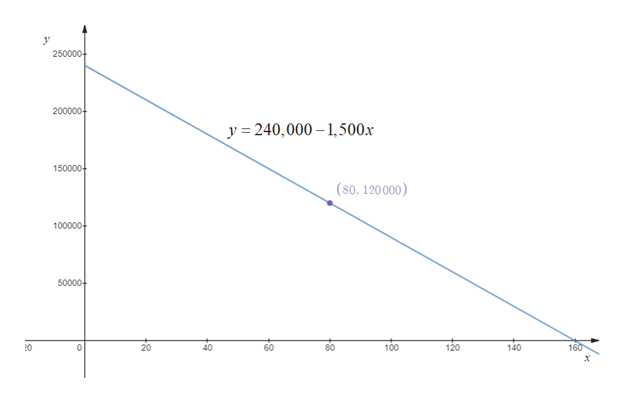A \$240,000 building is depreciated by its owner. The value y of the building after x months of use isy = 240,000 − 1500x.(a) Graph this function for x ≥ 0.  (b) How long is it until the building is completely depreciated (its value is zero)? months (c) The point (80, 120,000) lies on the graph. Explain what this means.The point (80, 120,000) means that after months the value of the building will be \$ .

Question

A \$240,000 building is depreciated by its owner. The value y of the building after x months of use is

y = 240,000 − 1500x.
(a) Graph this function for x ≥ 0.

(b) How long is it until the building is completely depreciated (its value is zero)?
months

(c) The point (80, 120,000) lies on the graph. Explain what this means.
The point (80, 120,000) means that after months the value of the building will be \$ .
Step 1

(a) The graph of the equation y = 240,000 – 1,500x for x ≥ 0 is shown in below figure.help_outlineImage Transcriptionclose250000 200000 y 240,000 , 500x 150000 (80, 120000) 100000 50000 80 100 120 140 160 60 20 40 fullscreen
Step 2

(b) The building is completely depreciated at x = 0.

From the figure in step 1, it is observed that the line y crosses x-axi...

Want to see the full answer?

See Solution

Want to see this answer and more?

Our solutions are written by experts, many with advanced degrees, and available 24/7

See Solution
Tagged in

Other